# Stacked Area Charts and Mathematical Approximations

### A review of the usefulness of communicating data via stacked area charts.

· Big Data Zone · Opinion
Save
2.65K Views

i've previously noted that i think stacked area charts are frequently used when a conventional line chart would be a better option. here is the (fictional) example i used previously and the conventional line chart alternative.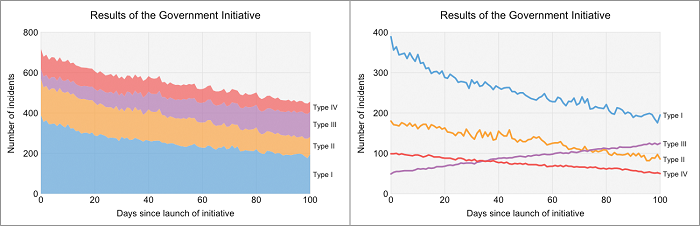in short, if you want people to be able to make reasonably accurate judgments of the magnitudes of the individual components, and how they change depending on some other variable (such as time), the conventional line chart design is almost always going to be the best option. the lack of a steady baseline for all but the bottom component makes this task difficult for the stacked area chart.

stacked area charts can be useful if you want to illustrate an ordered sum of components that change with another variable. while previously i suggested how the cost of milk production from farm to shop might change with time might be suitable, here i'd like to consider something very different: selected mathematical series.

you're probably familiar with trigonometric functions like sine and cosine and you may also know about the exponential function and hyperbolic functions . it's fairly easy to draw graphs of these functions if you have a calculator of some sort. when tied up in complicated equations, these functions may become awkward to deal with. consequently, alternative ways of approximating these functions can come in very handy.

the functions mentioned above are all analytic functions . what this really means is quite complicated to attempt to explain so i won't try to do so here. instead, i'll just stick to the following: these functions can all be written as a sum of powers of their argument (typically denoted x ), that is, as a polynomial . being explicit helps, so here is a way of rewriting the exponential function: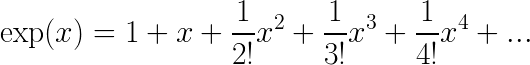in a similar manner, here is another way of expressing the cosine function: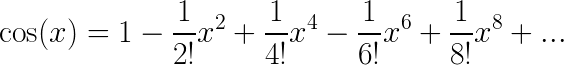and, here is the hyperbolic cosine function (typically written as cosh):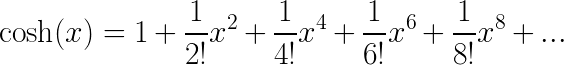in general, to get an exact value for one of these functions using summation, we need to sum to infinity. this is not the case at the origin where all but the first term will equal 0. close to the origin we will also get a good approximation as x is small. but, how close and how good? we can plot the first few terms of, for example, the exponential function expression and see. the black line in the gif below shows the exact exponential function, the blue wedges show the result of adding more and more terms from the right-hand side of the equation (from the zeroth power of x up to the 8th) for the exponential function above. the translucent red wedge indicates the area not covered by the polynomial approximation.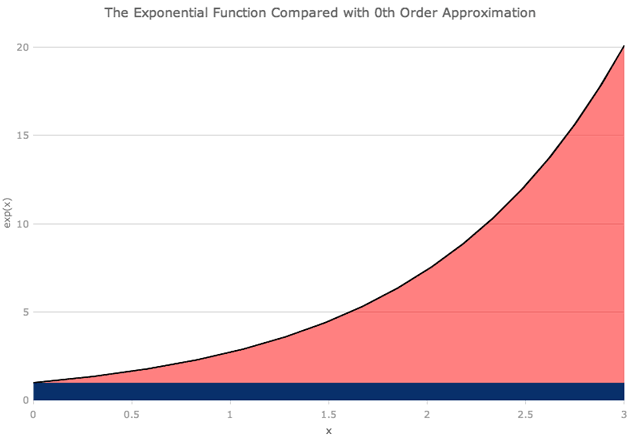below about x =1 we can see that the first three terms of the polynomial are a pretty good approximation for the exponential function. to get a good approximation around x =3 we need to go up to the sixth or seventh power of x (i.e. seven or eight terms of the polynomial). as the gif below shows, even going to the eighth power of x isn't sufficient around x =6.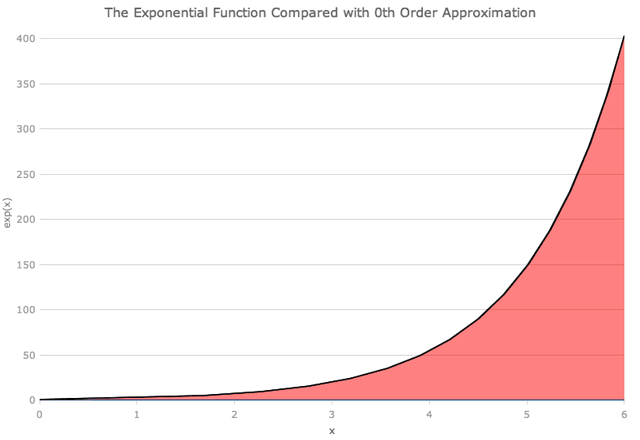we can look at the hyperbolic cosine function in a similar way, though there are no terms with odd powers of x .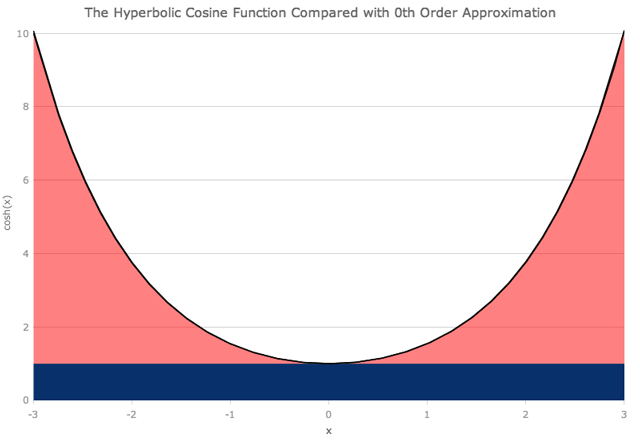as you might expect, when we look at large distances from the origin, we need more and more terms of the polynomial in order to closely match the exact function. at x =±6, adding up terms up to the 8th power of x is not sufficient to get a good approximation.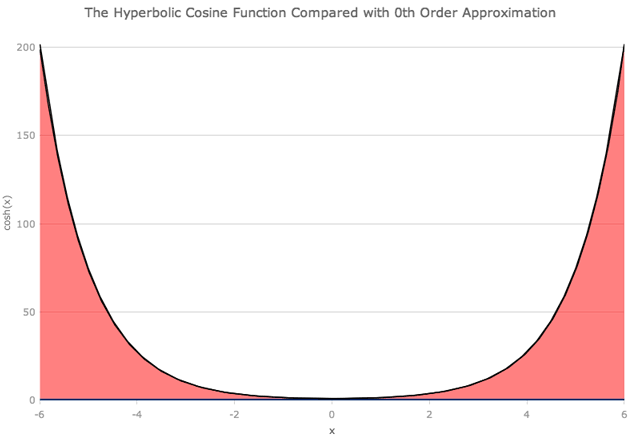i think these are cases where stacked area charts can be of real use. we're genuinely interested in the progressive sums of components, not the individual parts and that's where stacked charts excel.

you probably noticed that i skipped over producing charts for the cosine function. that's because stacked charts fail. why? because successive terms have opposite signs. while including more and more terms in the polynomial approximation does get you closer and closer to the exact function, you can't show this as a simple stack because some terms add to the total while others subtract. this also a problem for the exponential function when x is negative: terms involving even powers of x will be positive while those involving odd powers of x will be negative. this is a purely visual issue that doesn't crop up when we plot lines instead of stacks.

hopefully, i've shown that stacked area charts can be useful when it is the ordered sums of components that are of interest and if the conditions are right. for the conditions to be right then all components of the stack must share the same sign (or be 0) at each (visible) point along the horizontal axis.

Topics:
chart, graph, math, javascript

Published at DZone with permission of Josh Anderson, DZone MVB.

Opinions expressed by DZone contributors are their own.